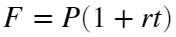# Problem 56075. Simple Interest : Calculate Future Value

Theorem : Simple Interestwhere;
• F : Future Value
• P : Present Value
• r : Annual simple interest rate (decimal)
• t : Time in years
Calculate F, given P, r and t. Round to nearest cent.

### Solution Stats

88.57% Correct | 11.43% Incorrect
Last Solution submitted on Oct 28, 2023

### Community Treasure Hunt

Find the treasures in MATLAB Central and discover how the community can help you!

Start Hunting!